# Electronics and Communication Engineering - Analog Electronics

26.

In figure base current is 10 μA and βdc = 100. Then VE =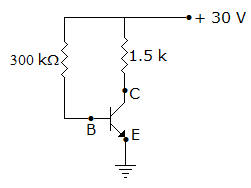A. 5 V B. 10 V C. 15 V D. 20 V

Explanation:

IC = 1 mA and VCE = 20 - 5 x 103 x 1 x 10-3 = 15 V.

27.

It has been found that in a rectifier circuit with RC filter one RC section reduces ripple by 15%. Two RC sections are used in cascade the reduction in ripple would be

 A. 15% B. 30% C. 150% D. 225%

Explanation:

One filter reduces ripple to 0.15 of initial value. The second filter reduces ripple to 0.15 x 0.15 of initial value.

28.

An op-amp has

 A. low input and output impedance B. low input impedance and high output impedance C. low output impedance and high input impedance D. high output impedance and high input impedance

Explanation:

High input impedance ensures minimum loading of source. Low output impedance is required for impedance matching at output.

29.

In the figure, assume the op-amp is to be ideal. The output Vo if the circuit is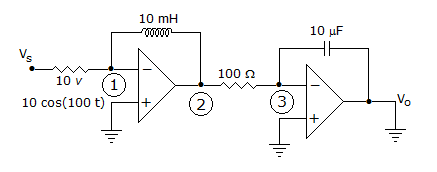A. 10 cos (100t) B.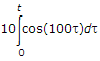C.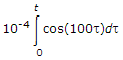D.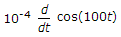Explanation:

KCL at mode one.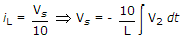... (1)

Applying KCL at node (3)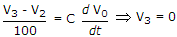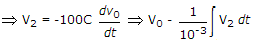... (2)

From (1)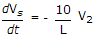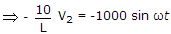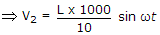V2 = 100 L sin ωt

Put value of V2 in equation (2) and solve for V0 .

30.

As the ratio Rf/RL increases the efficiency of a rectifier increases.

 A. True B. False

As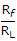increases efficiency decreases.